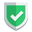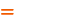我们相信：世界是美好的，你是我也是。平行空间的世界里面，不同版本的生活也在继续...

## 数组分割

``````_list = ["a","b","c","d","e"]
_list2 = _list[slice(2)] #["a","b"]
_list3 = _list[slice(1,2)] #["b"]
_list4 = _list[slice(3,5)] #["d","e"]
_list5 = _list[slice(9,10)] #[]
_list6 = _list[slice(4,10)] #["e"]``````

• 切片slice(N)，就返回第一个到第N个。
• 切片slice(N,M)，就返回第N+1个到第M个。越界的话，就返回空。一部分越界的话，就返回不越界的。

`php`中的类似函数是`array_slice`，范例如下：

``````\$input = array("a", "b", "c", "d", "e");
\$output = array_slice(\$input, 2);      // 返回 "c"， "d" 和 "e"
\$output = array_slice(\$input, -2, 1);  // 返回 "d"
\$output = array_slice(\$input, 0, 3);   // 返回 "a"， "b" 和 "c"``````

## 数组连接

``````_list = ["a","b"]
# _str = _list.join(",") # AttributeError: 'list' object has no attribute 'join'
_str =",".join(_list) # a,b``````

``AttributeError: 'list' object has no attribute 'join'``
`nodejs`中，是用`数组.join(字符串)`的，`php`中是使用`join(字符串,数组)`的。

## 字符串去除两边空白

`php`里面，使用`trim()``ltrim()``rtrim()`
`python`里面，函数名叫`strip()`，然后联动的函数叫做`lstrip()``rstrip()`

``_str=" a,b ".strip() # a,b``
`nodejs`里面没有内置的`trim()`，都是后来自定义的，暂不提。

## 结束语如果本文对您有帮助，或者节约了您的时间，欢迎打赏瓶饮料，建立下友谊关系。本博客不欢迎：各种镜像采集行为。请尊重原创文章内容，转载请保留作者链接。【福利】 腾讯云最新爆款活动！1核2G云服务器首年50元！【源码】本文代码片段及相关软件，请点此获取更多信息【绝密】秘籍文章入口，仅传授于有缘之人python